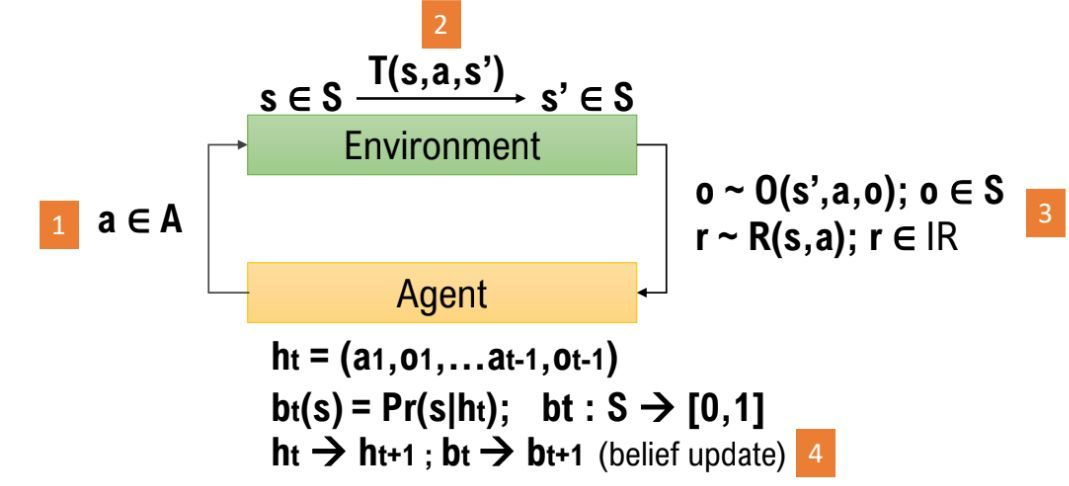# Design Principles¶

1. Distributions are the fundamental building blocks of a POMDP.

Essentially, a POMDP describes the interaction between an agent and the environment. The interaction is formally encapsulated by a few important generative probability distributions. The core of pomdp_py is built around this understanding. The interfaces in pomdp_py.framework.basics convey this idea. Using distributions as the building block avoids the requirement of explicitly enumerating over $$S,A,O$$.

1. POMDP = agent + environment

Like above, the gist of a POMDP is captured by the generative probability distributions including the TransitionModel, ObservationModel, RewardModel. Because, generally, $$T, R, O$$ may be different for the agent versus the environment (to support learning), it does not make much sense to have the POMDP class to hold this information; instead, Agent should have its own $$T, R, O, \pi$$ and the Environment should have its own $$T, R$$. The job of a POMDP is only to verify whether a given state, action, or observation are valid. See Agent and Environment.A Diagram for POMDP $$\langle S,A,\Omega,T,O,R \rangle$$. (correction: change $$o\in S$$ to $$o\in\Omega$$)